Factoring Practice - Level A Terms of Use    Contact Person: Donna Roberts1.
What is the greatest common factor of 32 and 64?

Choose:
 6 16 32 642.
What is the least common multiple of 6 and 9?

Choose:
 15 18 54 90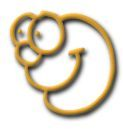3.
Two whole numbers have a least common multiple of 24. Each of the numbers is less than or equal to 12, and the greatest common factor of the two numbers is 4. What are the two numbers?

Choose:
 4 and 12 6 and 12 8 and 12 9 and 124. Which of the following expressions is(are) equivalent to 4(m + 5)?(Check all that apply, and hit SUBMIT!) 4m + 5 4m + 20 2m + 2 + 2m + 3 3(m + 8) + (m - 4) 2(m + 8) + m5.
Which list shows all of the factors of 54?

Choose:
 1, 2, 3, 4, 6, 9, 14, 18, 27, 54 1, 2, 3, 4, 6, 7, 8, 9, 14, 18, 27, 54 1, 2, 4, 6, 9, 14, 27, 54 1, 2, 3, 6, 9, 18, 27, 546.
For which pair of numbers is their least common multiple the same as their product?

Choose:
 2 and 8 6 and 9 5 and 7 5 and 107. Which of the following choices can represent 12 • 5 ? (Check all that apply, and hit SUBMIT!) 12 + 12 + 12 + 12 + 12 (3 • 4) • 5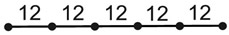length = ? (5 • 10) + (5 • 2) 12 • 12 • 12 • 12 • 12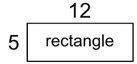area = ? 5(10 + 2)perimeter = ?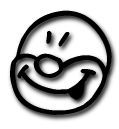8.
What is the least common multiple of 3, 8 and 12?

Choose:
 12 24 36 96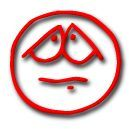9.
How many of the factors of 50 are prime numbers?

Choose:
 1 2 3 none10.
Factor completely: 32a - 40

Choose:
 2(16a - 20) 4(8a - 10) 4(9a - 10) 8(4a - 5)